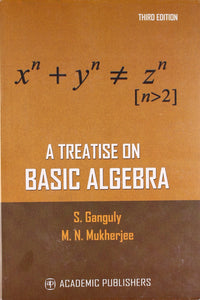a treatise on basic algebra -by sobhakar Ganguly

• Rs. 195.00
• Save Rs. 30.00

• designed for undergraduate students. • Contains complex numbers, polynomial equations, determinant and matrices, sets, relation and mapping, groups, rings, fields, vector space, eigen values, eigen vectors, quadratic forms etc. • Problems selected meticulously.
xx